# SSC JE Electrical Important MCQ Part 28

SSC JE Electrical Important MCQ PDF Part 28

1.The variable resistor R in Fig. is adjusted until it absorbs the maximum power from the circuit.What will be the value of R for maximum power.A. 25 Ω

B. 50 Ω

C. 15 Ω

D. 125 Ω

Answer : A (For maximum power- Source resistance=Load resistance or  Thevenin equivalent RTh=R2.Value of io in the circuit in Fig. is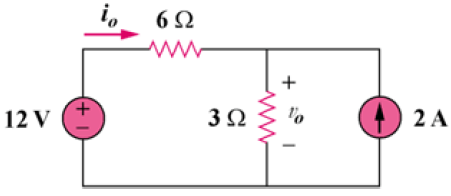A. 66.67 mA

B. 666.7 mA

C. 6.667 mA

D. 6667 mA

Answer : B (To get io, transform the current sources as shown in Fig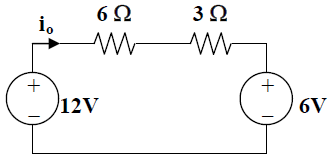From above Fig. -12 + 9io + 6 = 0

io=6/9

io = 666.7 mA)

3.A series RC circuit has ∣VR∣ = 12 V and ∣VC∣ = 5 V.The magnitude of the supply voltage is

A. -7 V

B. 7 V

C. 13 V

D. 17 V

Answer : C (In a series RC circuit, the formula for Vrms is given as

Vrms = √Vr² + Vc²,Vrms = √12² + 5²= √144+25 = √169= 13 V)

Electrical One Year Test Series Pass-Daily Test Plus Weekly Full Test

P → wind farms
Q → run-of-river plants
R → nuclear power plants
S → diesel power plants

A. P, Q & S only

B. P, R & S only

C. P, Q & R only

D. Q and R only

Answer : C (A base load power plant is a power station that usually provides a continuous supply of electricity throughout the year.Base Load power plants are (i) Wind farms,(ii) Run of river plants,(iii) Nuclear power plants,(iv) Geothermal power plant,(v) Coal-fired power plant,(vi) Tidal power plant)

5.If the inductance and capacitance of a system are 1·0 H and 0·01 μF respectively and the instantaneous value of current interrupted is 10 amp, the voltage across the breaker contacts will be __________ kV

A. 101

B. 100

C. 99

D. 9.9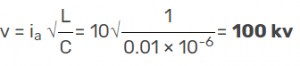6.A power system network with the capacity at 100 MVA has a source impedance at 10% at a point. The fault at that point is __________ MVA

A. 1000 MVA

B. 10.7 MVA

C. 10.1MVA

D. 10.00 MVA

Answer : A (Fault MVA =Capacity/% of reactance=100/0.10= 1000 MVA)

7.The per unit impedance of a circuit element is 0.15. If the base kV and base MVA are halved, then the new value of the per unit impedance of the circuit element will be

A. 30.3

B. 0.030

C. 0.30

D. 30Electrical One Year Test Series Pass-Daily Test Plus Weekly Full Test

8.A consumer consumes 600 kWh per day at a load factor of 0.40. If the consumer increases the load factor of 0.70 without increasing the maximum demand. The consumption of energy in kWh is______

A. 1050 kWh

B. 1500 kWh

C. 1005 kWh

D. 5010 kWh

Answer : A (Load factor =Energy consumed in 24 hours/Maximum demand in kW × 24

or 0.40 =600/Maximum demand in kW × 24

Maximum demand in kW =600/24 × 0.40= 62.5 kW

Energy consumed in 24 hours = (Load factor) × (maximum demand in kW) × 24

= 0.70 × 62.5 kW × 24 = 1050 kWh)

9.To limit current chopping in vacuum circuit breakers, the contact material used has

A. high vapour pressure and low conductivity properties

B. high vapour pressure and high conductivity properties

C. low vapour pressure and high conductivity properties

D. low vapour pressur e and low conductivity properties

10.The current chopping tendency is minimised by using the SF6 gas at relatively

A. High pressure and low velocity

B. High pressure and high velocity

C. Low pressure and low velocity

D. Low pressure and high velocity.

11. A shunt generator delivers 195 A at terminal p.d. of 250 V. The armature resistance and shunt field resistance are 0.02 Ω and 50 Ω respectively.What will be E.M.F. generated

A. 254 V

B. 250 V

C. 246 V

D. 255 V

Answer : A (Ish = 250/50 = 5 A ; Ia = 195 + 5 = 200 A

Armature voltage drop = IaRa = 200 × 0.02 = 4 V

∴ Generated e.m.f. = 250 + 4 = 254 V)

12. A 4-pole, 900 r.p.m. D.C. machine has a terminal voltage of 220 V and an induced voltage of 240 V at rated speed. The armature circuit resistance is 0.2 Ω.What will be the armature current Ia

A. 200 A

B. 100 A

C. 50 A

D. 25 A

Answer : B (E − V = IaRa or 240 − 220 = Ia × 0.2  or Ia = 100 A)

13. A 5-H inductor changes its current by 3 A in 0.2 s. The voltage produced at the terminals of the inductor is

A. 75 V

B. 8.888 V

C. 3 V

D. 1.2 V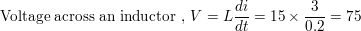14.In below waveform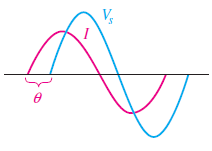B. I is in phase with Vs

C. I lags Vs

D. None of these

Answer : A (I leads Vs because I attending maximum and minimum value before V,Also I started θ before V)

Electrical One Year Test Series Pass-Daily Test Plus Weekly Full Test

15.For the circuit in Figure,The impedance (Z) at the resonance frequency (fr) is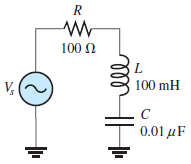A. 3.95 kΩ

B. 100 Ω

C. 100 kΩ

D. 2.53 kΩ

Answer : B (At the fr impedance is equal to R.So Z=R=100 Ω)

16.For the circuit shown in Fig, Value of  VCE isA. -5 V

B. 5 V

C. 6 V

D. -6 V

Answer : A (Consider the two battery circuits of Fig. separately.

Current in the 20 V battery circuit ABCD is 20 (6 + 5 + 9) = 1A.

Similarly,current in the 40 V battery circuit EFGH is = 40/(5 + 8 + 7) = 2A.

For finding VCE i.e. voltage of point C with respect to point E, we will start from point E and go to C via points H and B VCE = (− 5 × 2) + (10) − (5 × 1) = − 5V)

17.Which of Diode symbol have not correctly matchedA. A

B. B

C. C

D. D

Answer : D (Symbol D is a symbol of TRIAC)

18.Circuit shown in figure is a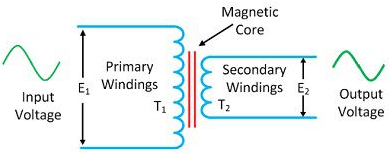A. Step-up Transformer

B. Step-down Transformer

C. Isolation Transformer

D. Auto Transformer

Answer : B (The number of turns on the primary of the transformer is greater than the turn on the secondary of the transformer, i.e., T2 < T1. So it is a step-down transformer.)

19.A load is connected to a network. At the terminals to which the load is connected, RTh = 10 Ω and VTh = 40 V. The maximum possible power supplied to the load is

A. 160 W

B. 80 W

C. 40 W

D. 1 W

Answer : C (Pmax=VTH2/4RTH (As per Maximum Power Theorem)=402/4*10=1600/40=40 W)

20.The reciprocal of resistance is

A. voltage

B. current

C. conductance

D. coulombs

Answer : C (Conductance is inversely related to resistance. If R is the resistance of a component or device (in ohms), then the conductance G (in siemens) is given by G = 1/R)